# Initial value problem

## Definition

An initial value problem in the context of a differential equation (here, an ordinary differential equation) is the following data:

• A differential equation$F(x,y,y',\dots,y^{(k)}) = 0$ (the independent variable here is$x$ and the dependent variable is$y$). This is a differential equation of order$k$.
• A tuple of numbers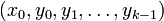$(x_0,y_0,y_1,\dots,y_{k-1})$

### Solution concept

A solution to this is a functional (or relational) solution to the original differential equation such that at$x = x_0$, we have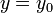$y = y_0$ and the$i^{th}$ derivative is$y_i$ for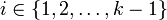$i \in \{ 1,2,\dots,k-1\}$. More concretely, a function$f$ solves the initial value problem if it solves the differential equation and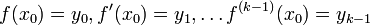$f(x_0) = y_0, f'(x_0) = y_1, \dots f^{(k-1)}(x_0) = y_{k-1}$, i.e.,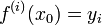$f^{(i)}(x_0) = y_i$ for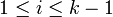$1 \le i \le k - 1$.

### Intuition behind going one derivative less

Note that the typical setup of an initial value problem specifies derivatives only up to the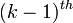$(k-1)^{th}$ for an order$k$ differential equation. The intuition is that the differential equation controls the$k^{th}$ (and higher) derivatives in terms of the derivatives up to the$(k-1)^{th}$. In the case of an explicit differential equation, the$k^{th}$ and higher derivatives are uniquely determined. In general, they are usually determined up to a finite or discrete ambiguity.

## Solution strategy

### In terms of the general solution

The typical solution strategy for an initial value problem is as follows:

• First, find the general solution. For a differential equation of order$k$, we typically expect this to involve$k$ free parameters.
• Plug in the conditions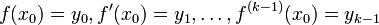$f(x_0) = y_0, f'(x_0) = y_1, \dots, f^{(k-1)}(x_0) = y_{k-1}$. These are$k$ conditions. Each condition gives an equation involving the$k$ free parameters. This gives a system of$k$ equations in$k$ variables.
• Solve the system. Assuming consistent and irredundant equations, we expect the solution set to be zero-dimensionsal, which typically means it is finite or discrete. In good situations, we may get a unique solution.
• Having found the solutions for the free parameters, plug these in to get the functional (or relational) solutions to the initial value problem.

### Other strategies

There are some strategies to solve initial value problems that do not rely on finding the general solution. Generally, these involve doing definite instead of indefinite integrations in the process of finding the solution. This way we avoid introducing unnecessary constants. In some situations, this can save us from the effort of making cases based on the signs of those unknown constants, because definite integration avoids the introduction of unnecessary parameters.

## Variations

Instead of a single initial value problem, we may be given the values of the function and some of its derivatives at multiple points. The trade-off is that fewer derivatives may be specified. The solution method is the same: find the general solution, then plug in the conditions to get equations for the free parameters. In general, we would like to have$k$ conditions in total (values of functions and derivatives) in order to get a unique, or finite, set of possible solutions.

One extreme case of a multiple initial value problem is the case where the value of the function (but no derivatives) is specified at$k$ distinct points of the domain.

## Examples

### Example of a separable differential equation

This is a first-order differential equation, so there will be one free parameter, and the initial value specification only involves specifying the function value (no derivatives) at one point.

Consider the separable differential equation: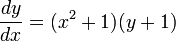$\frac{dy}{dx} = (x^2 + 1)(y + 1)$

This has a stationary solution$y = -1$. The relational solution family is given by: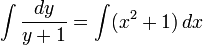$\int \frac{dy}{y + 1} = \int (x^2 + 1) \, dx$

Integrating:$\ln|y + 1| = \frac{x^3}{3} + x + C$

Exponentiate:$|y + 1| = e^C \exp\left(\frac{x^3}{3} + x \right)$

Let$k = \operatorname{sgn}(y + 1)e^C$ and get (NOTE: This$k$ has nothing to do with the$k$ denoting the order of a differential equation, used elsewhere on this page):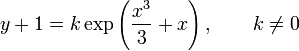$y + 1 = k \exp\left(\frac{x^3}{3} + x \right), \qquad k \ne 0$

So:$y = k \exp\left(\frac{x^3}{3} + x \right) - 1, \qquad k \ne 0$

We can combine back the stationary solution by allowing$k = 0$ to get: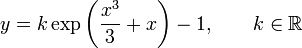$y = k \exp\left(\frac{x^3}{3} + x \right) - 1, \qquad k \in \R$

We can see from this that every initial value specification yields a unique value of$k$ (we essentially get a linear equation in$k$), and hence every initial value problem for this differential equation has a unique solution.

Consider two different initial value problems associated with this differential equation:

•$x = 1, y = 2$: In this case, we get:$2 = ke^{4/3} - 1 \implies 3 = ke^{4/3} \implies k = 3e^{-4/3}$

Plug back into the original to get the unique solution to the initial value problem: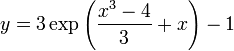$y = 3 \exp\left(\frac{x^3 - 4}{3} + x \right) - 1$

We can verify that this is both a solution to the differential equation and satisfies the initial value condition.

•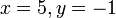$x= 5, y = -1$: In this case, we get: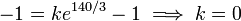$-1 = ke^{140/3} - 1 \implies k = 0$

So we get the stationary solution$y = -1$.

This is as expected -- for any initial value condition where the initial value of$y$ is a stationary solution constant, the stationary solution should be one solution to the initial value problem. In our case, every initial value problem has a unique solution, so it is the only solution.